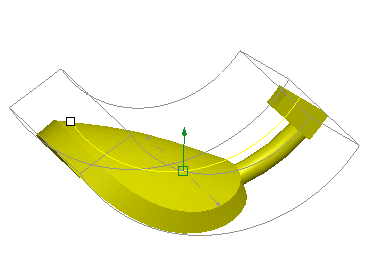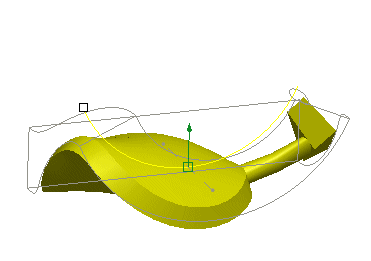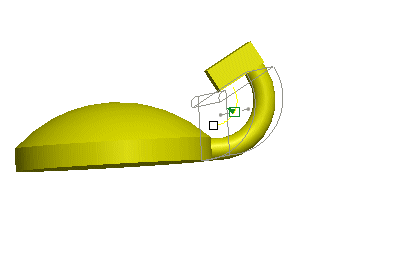Surfacing > Warp > Bend Operation > Example: The Bend Operation
Example: The Bend Operation
The following figure shows a Warp model and the Bend marquee.1. The Bend marquee
The following figure shows the model after a Bend operation.The following figure shows the model after a Bend operation with depth applied.The following figure shows the model after a Bend operation on a section defined by customizing the marquee.